#### Formulating the problem

Let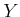denote the observation space, which is the set of all possible observations,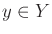. For convenience, suppose that,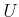, and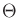are all discrete. It will be assumed as part of the model that some constraints on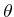are known once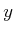is given. Under the nondeterministic model a set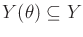is specified for every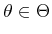. The set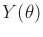indicates the possible observations, given that the nature action is. Under the probabilistic model a conditional probability distribution,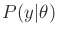, is specified. Examples of sensing models will be given in Section 9.2.4. Many others appear in Sections 11.1.1 and 11.5.1, although they are expressed with respect to a state spacethat reduces toin this section. As before, the probabilistic case also requires a prior distribution,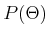, to be given. This results in the following formulation.

Formulation 9..5 (A Game Against Nature with an Observation)
1. A finite, nonempty setcalled the action space. Each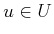is referred to as an action.
2. A finite, nonempty setcalled the nature action space.
3. A finite, nonempty setcalled the observation space.
4. A setor probability distributionspecified for every. This indicates which observations are possible or probable, respectively, ifis the nature action. In the probabilistic case a prior,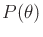, must also be specified.
5. A function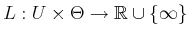, called the cost function.

Consider solving Formulation 9.5. A strategy is now more complicated than simply specifying an action because we want to completely characterize the behavior of the robot before the observation has been received. This is accomplished by defining a strategy as a function,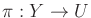. For each possible observation,, the strategy provides an action. We now want to search the space of possible strategies to find the one that makes the best decisions over all possible observations. In this section,is actually a special case of an information space, which is the main topic of Chapters 11 and 12. Eventually, a strategy (or plan) will be conditioned on an information state, which generalizes an observation.

Steven M LaValle 2020-08-14